# Geometry Midterm Exam Chapter One Chapter Two Chapter

• Slides: 52Geometry – Midterm Exam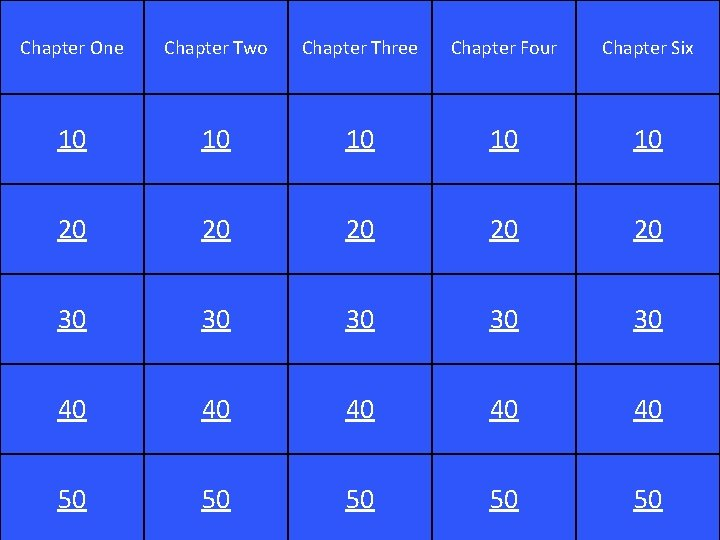Chapter One Chapter Two Chapter Three Chapter Four Chapter Six 10 10 10 20 20 20 30 30 30 40 40 40 50 50 50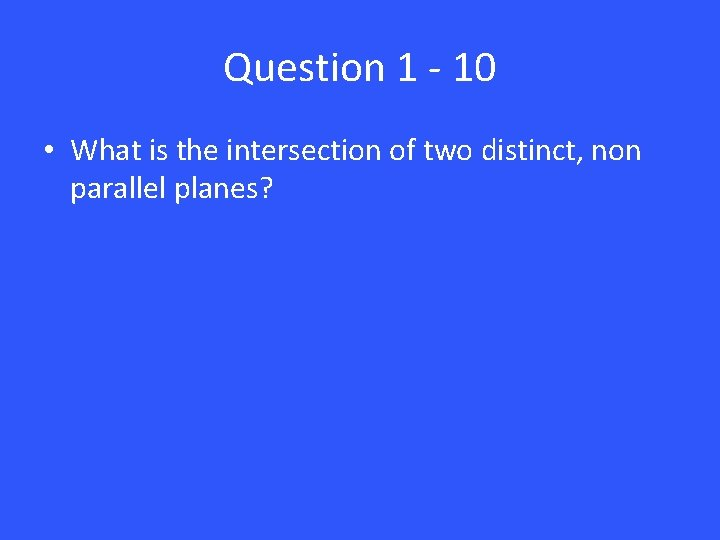Question 1 - 10 • What is the intersection of two distinct, non parallel planes?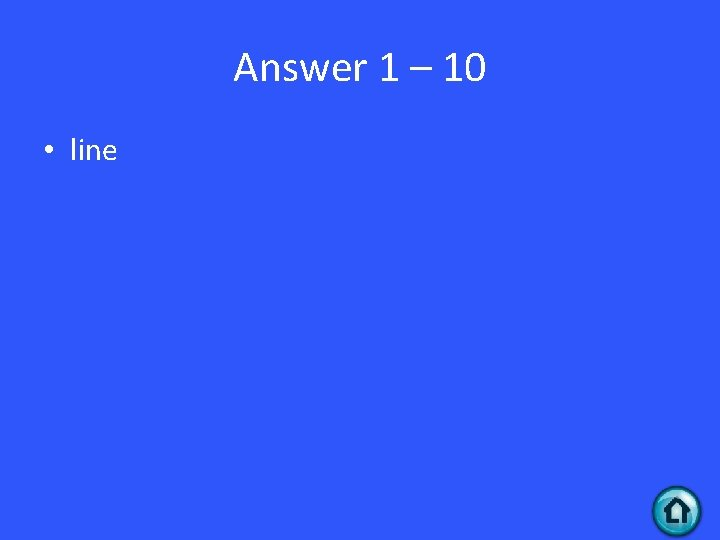Answer 1 – 10 • lineQuestion 1 - 20 • Point S lies between R and t on segment RT. If RS = 8 y + 4, ST = 4 y+8, and RT = 15 y – 9, find the value of y. Then find RS, ST, and RT.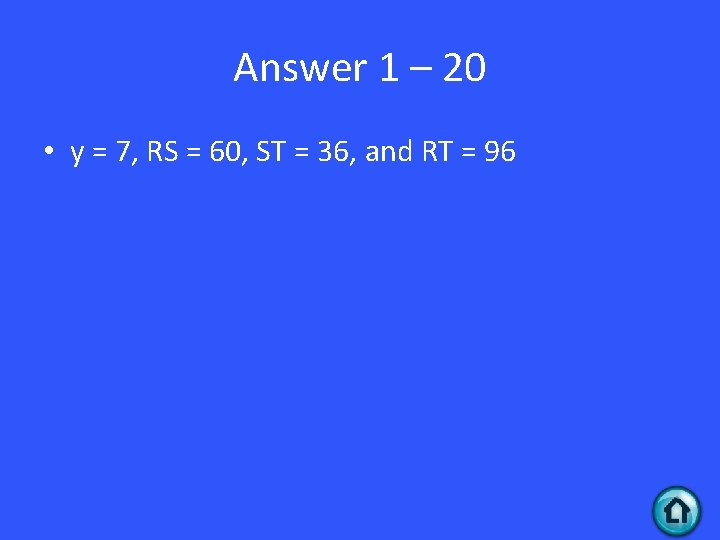Answer 1 – 20 • y = 7, RS = 60, ST = 36, and RT = 96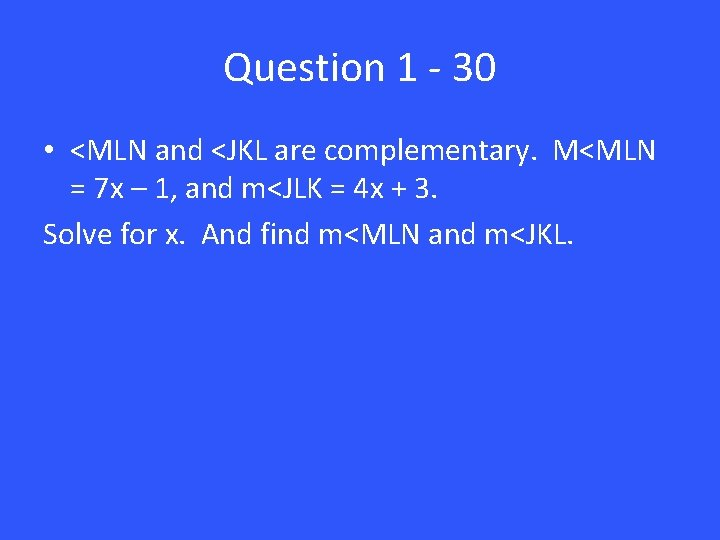Question 1 - 30 • <MLN and <JKL are complementary. M<MLN = 7 x – 1, and m<JLK = 4 x + 3. Solve for x. And find m<MLN and m<JKL.Answer 1 – 30 • X = 8, m<MLN = 55 and m<JKL = 35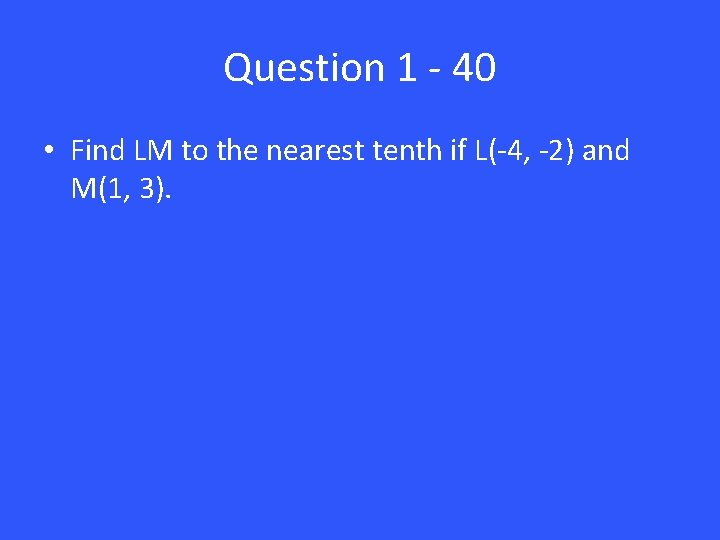Question 1 - 40 • Find LM to the nearest tenth if L(-4, -2) and M(1, 3).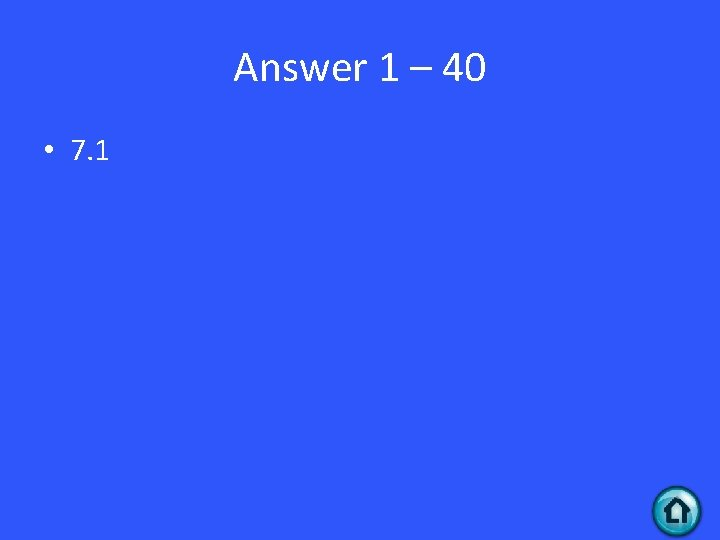Answer 1 – 40 • 7. 1Question 1 - 50 • Ray SV bisexts <RST. If <RSV = 60, what is m<RST?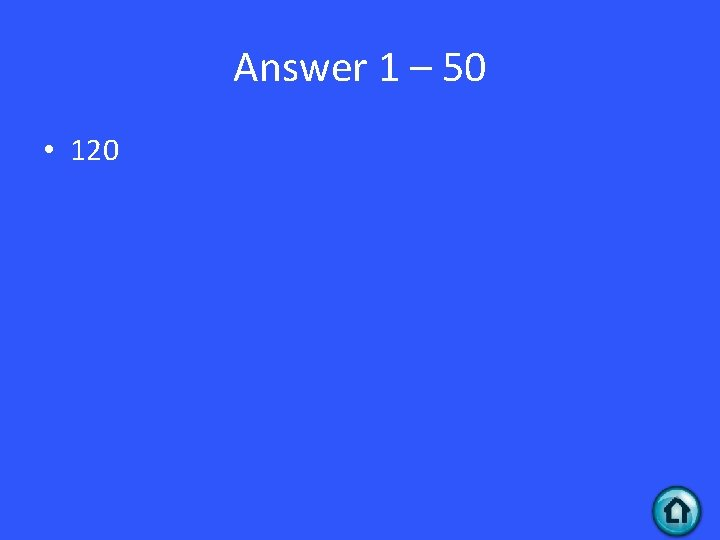Answer 1 – 50 • 120Question 2 - 10 • Find a counterexample to prove this statement false. The cube of a number is always greater than that number.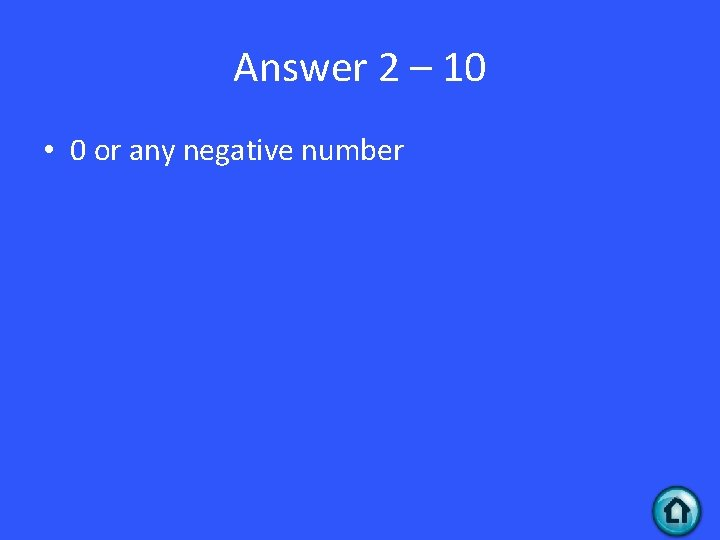Answer 2 – 10 • 0 or any negative numberQuestion 2 - 20 • What does it mean to be a good definition.Answer 2 – 20 • clear and reversible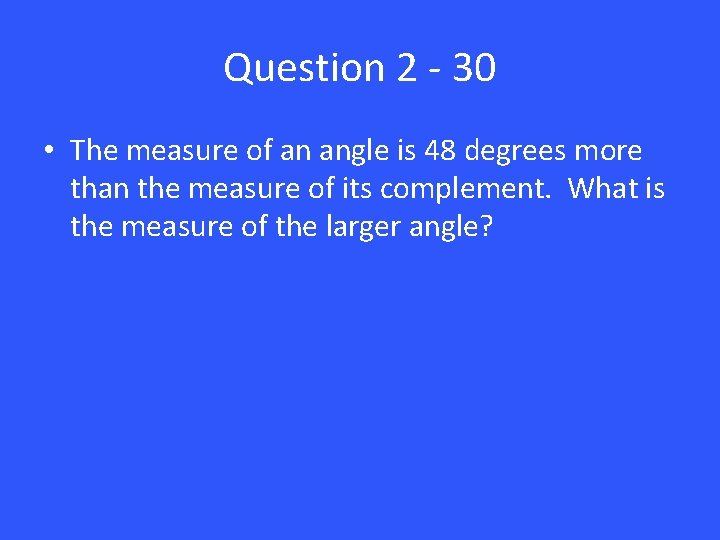Question 2 - 30 • The measure of an angle is 48 degrees more than the measure of its complement. What is the measure of the larger angle?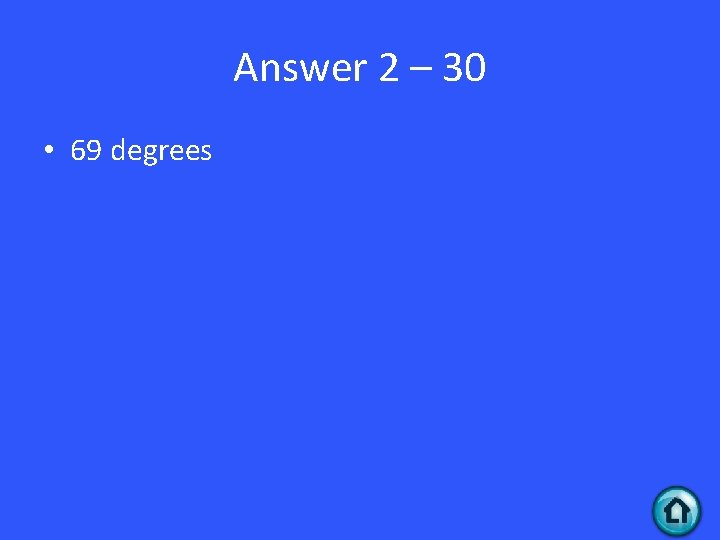Answer 2 – 30 • 69 degrees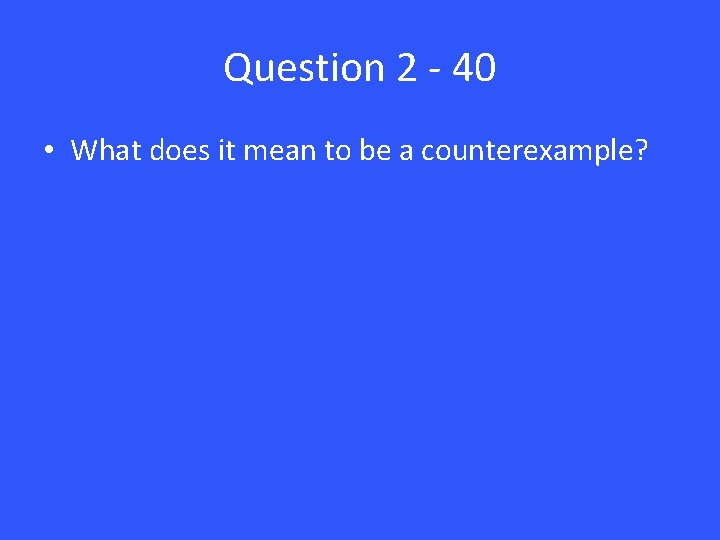Question 2 - 40 • What does it mean to be a counterexample?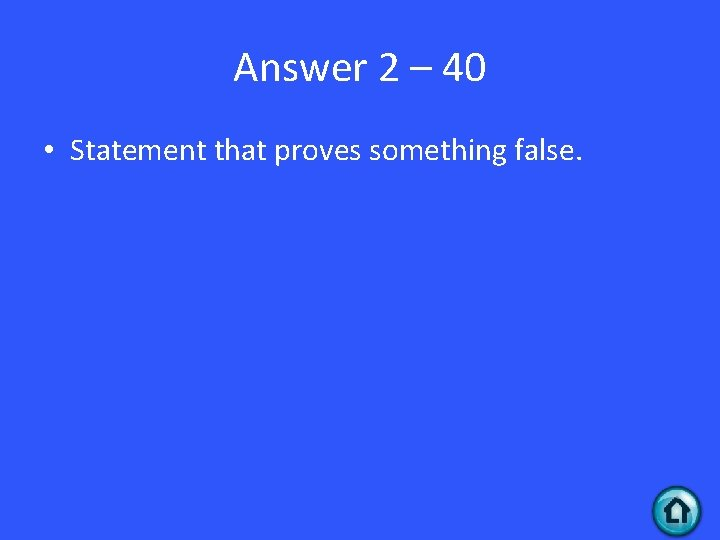Answer 2 – 40 • Statement that proves something false.Question 2 - 50 • Find the values of the variables.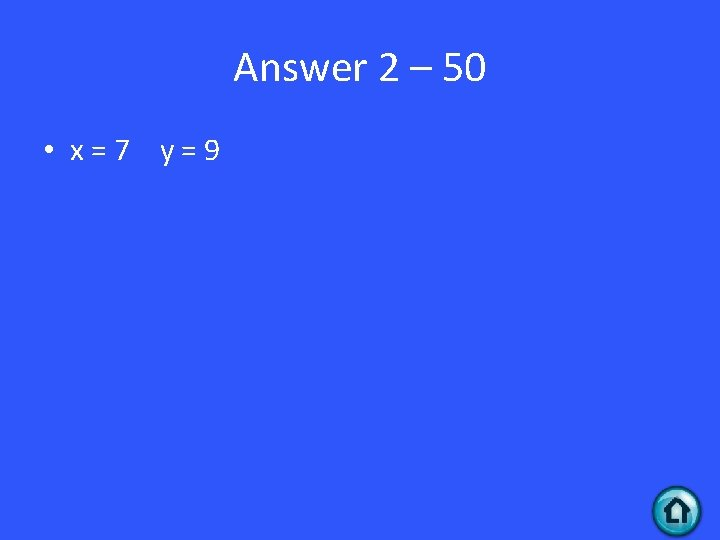Answer 2 – 50 • x=7 y=9Question 3 - 10 • Find the value of x and y:Answer 3 – 10 • x = 68 y = 32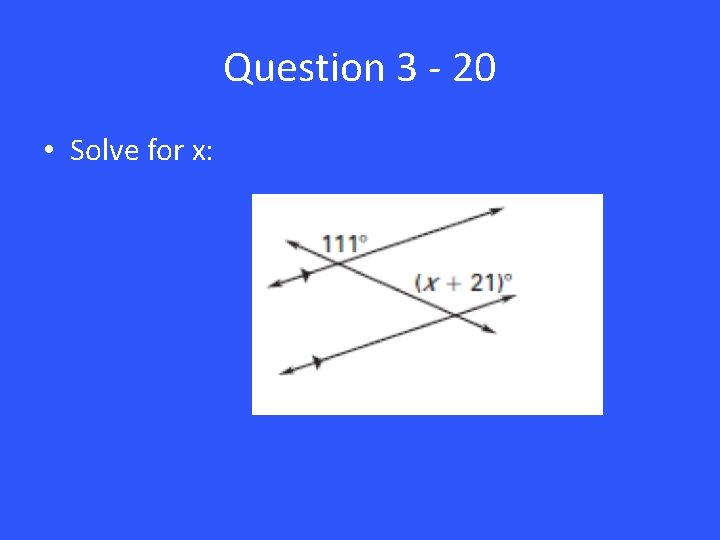Question 3 - 20 • Solve for x: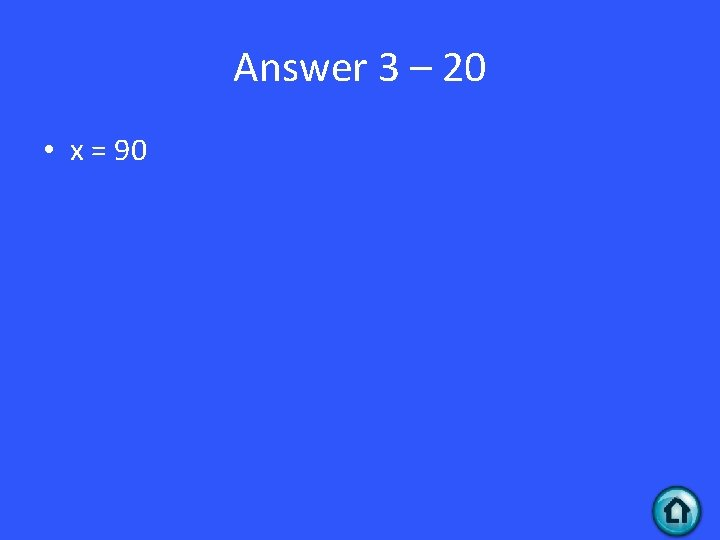Answer 3 – 20 • x = 90Question 3 - 30 • Find the equation of the line that is perpendicular to y = 1/2 x – 5 going through the coordinate point (4, 0)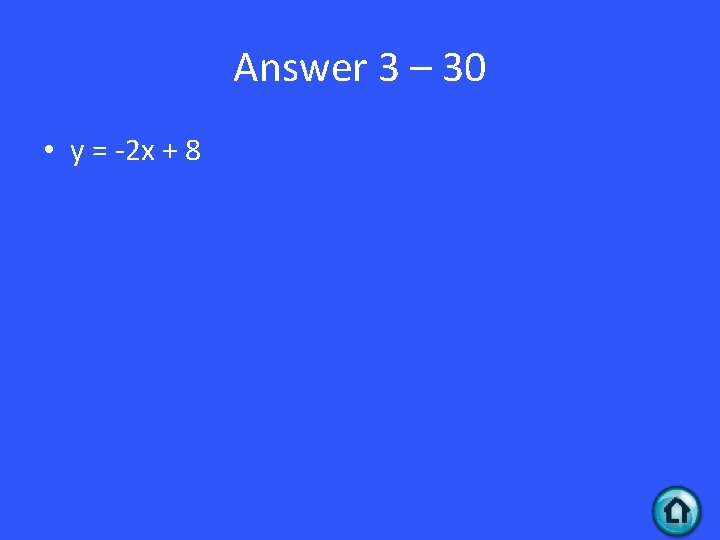Answer 3 – 30 • y = -2 x + 8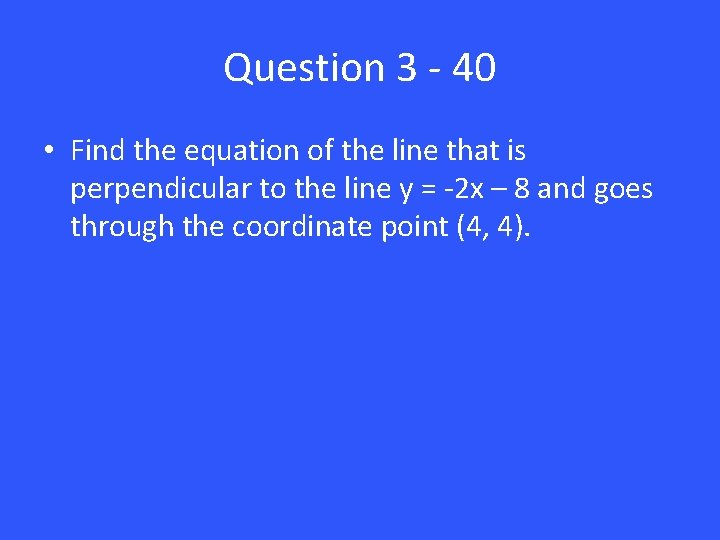Question 3 - 40 • Find the equation of the line that is perpendicular to the line y = -2 x – 8 and goes through the coordinate point (4, 4).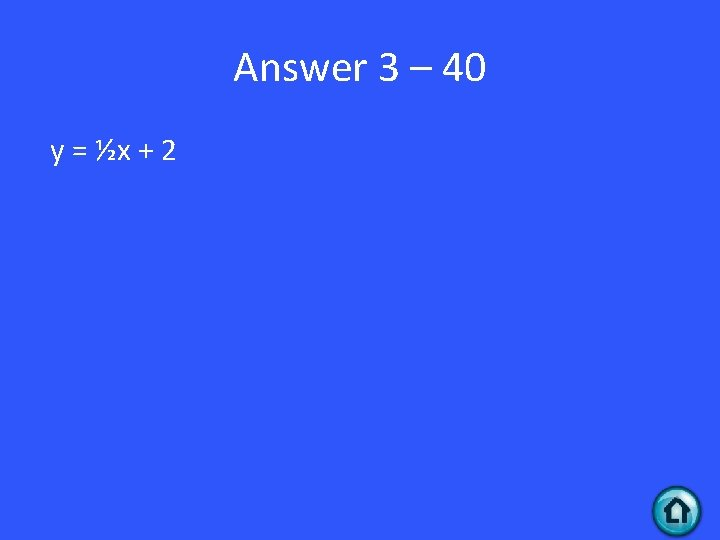Answer 3 – 40 y = ½x + 2Question 3 - 50 • Tell whether the lines through the given points are parallel, perpendicular, or neither. Line 1: (-9, 3) and (-5, 7) Line 2: (-11, 6) and (-7, 2)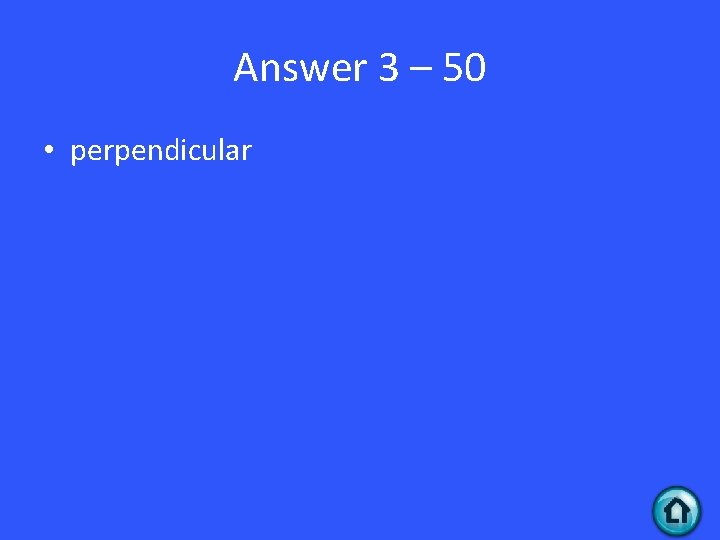Answer 3 – 50 • perpendicular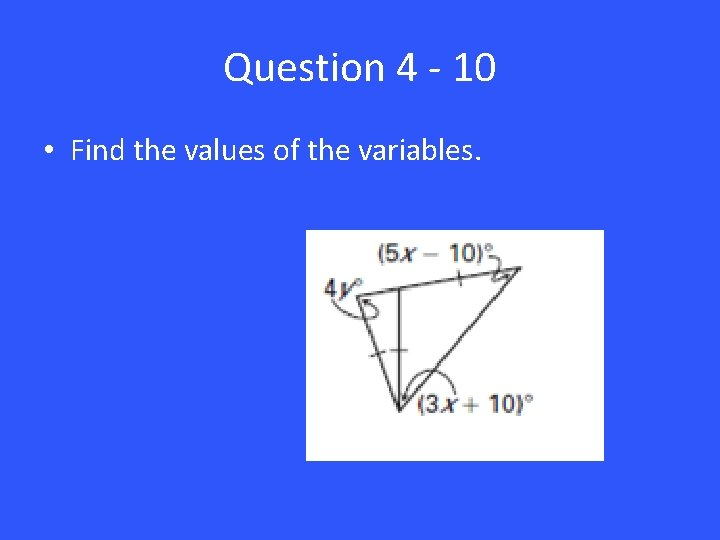Question 4 - 10 • Find the values of the variables.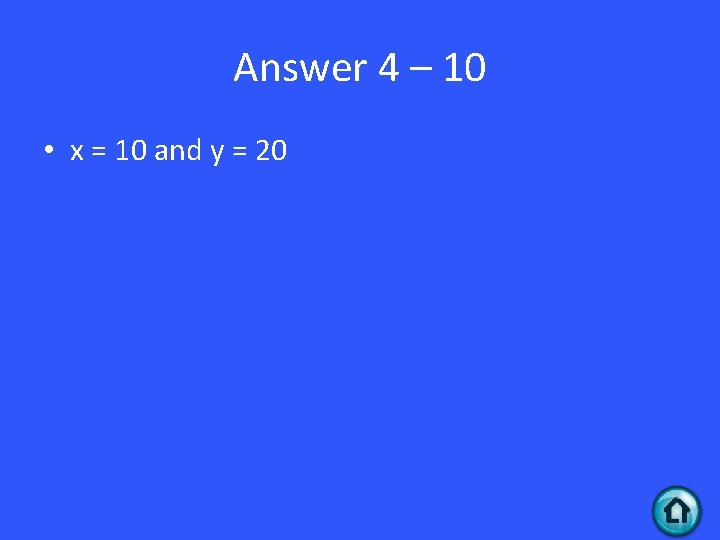Answer 4 – 10 • x = 10 and y = 20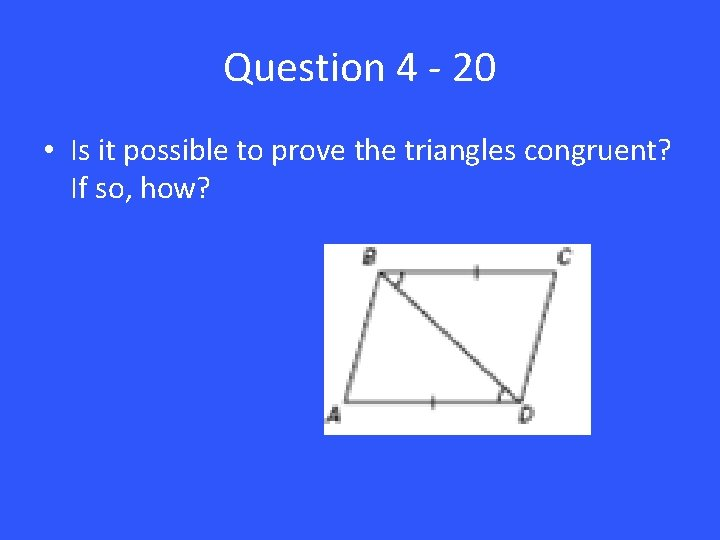Question 4 - 20 • Is it possible to prove the triangles congruent? If so, how?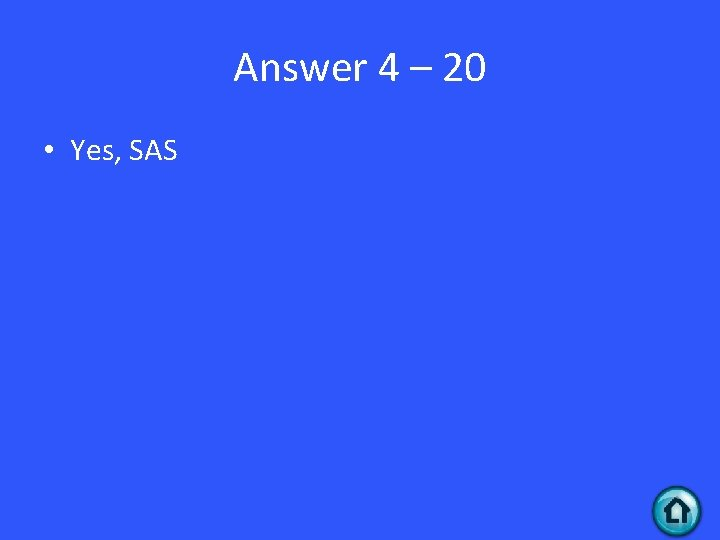Answer 4 – 20 • Yes, SASQuestion 4 - 30 • Is it possible to prove the triangle congruent? If so, how?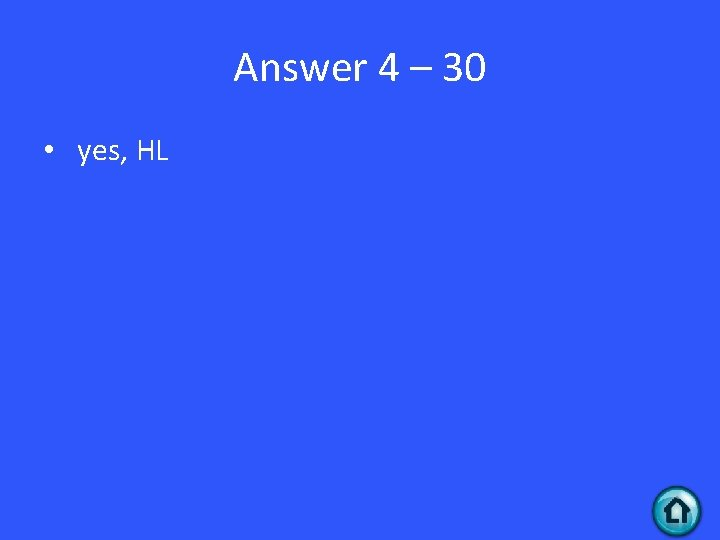Answer 4 – 30 • yes, HLQuestion 4 - 40 • Write an equation of the line that passes through (-2, 1) and is parallel to 10 x + 4 y = -8Answer 4 – 40 • y = -5/2 x – 4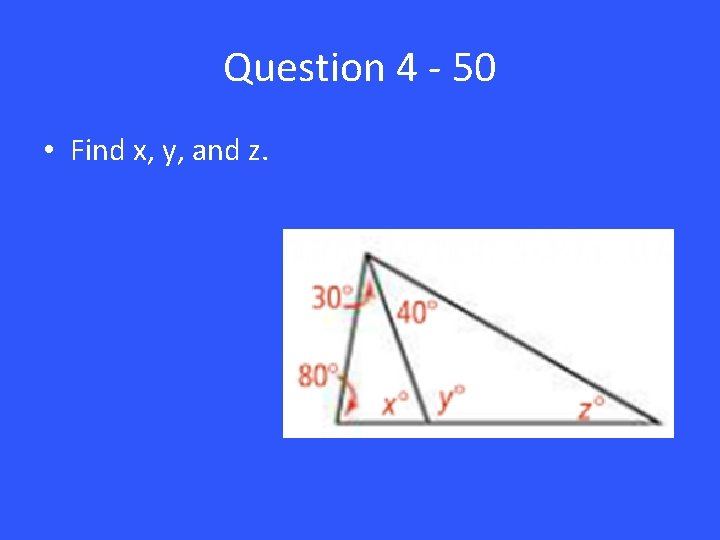Question 4 - 50 • Find x, y, and z.Answer 4 – 50 • x = 70, y = 110, z = 30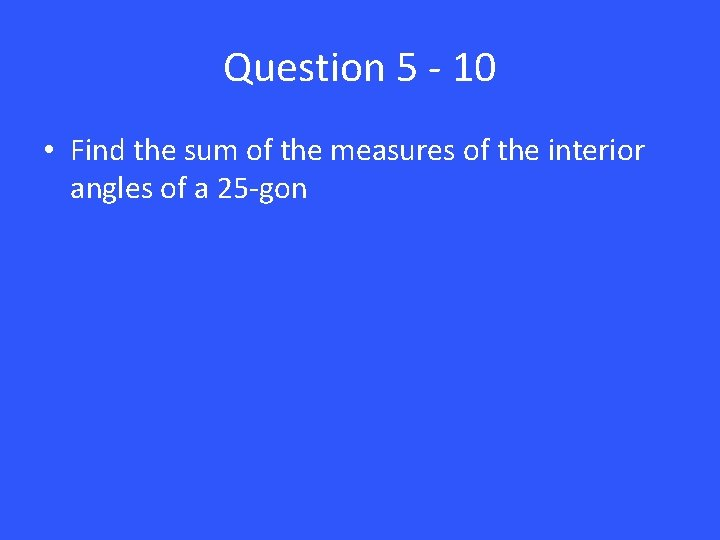Question 5 - 10 • Find the sum of the measures of the interior angles of a 25 -gonAnswer 5 – 10 • 4140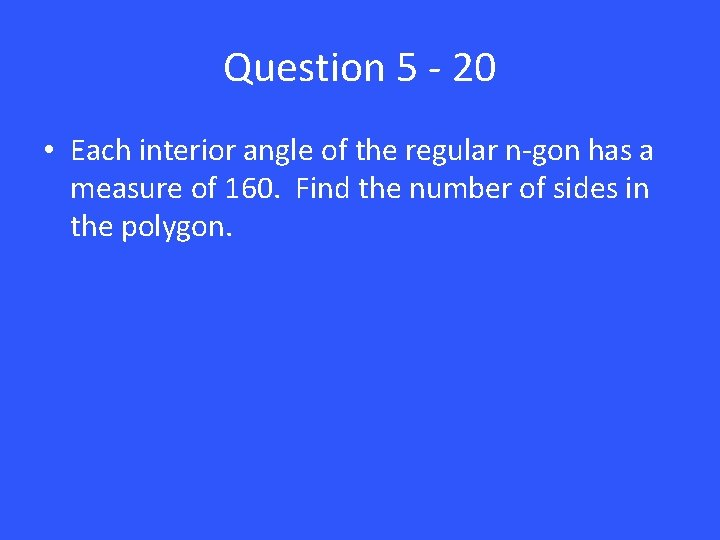Question 5 - 20 • Each interior angle of the regular n-gon has a measure of 160. Find the number of sides in the polygon.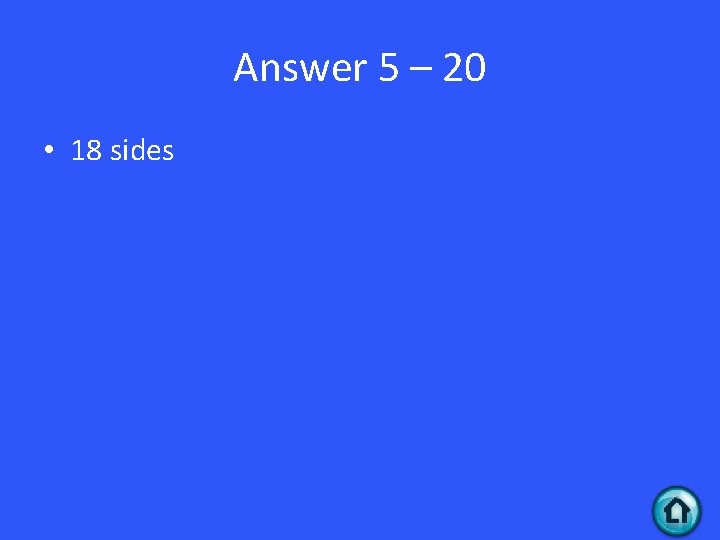Answer 5 – 20 • 18 sides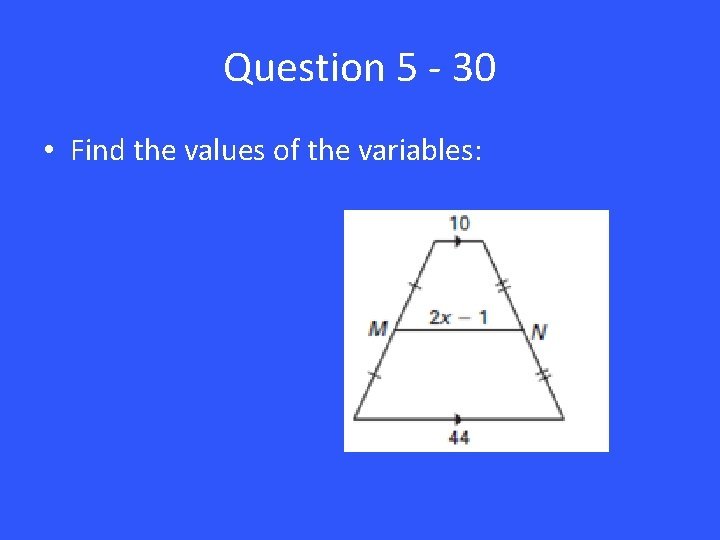Question 5 - 30 • Find the values of the variables: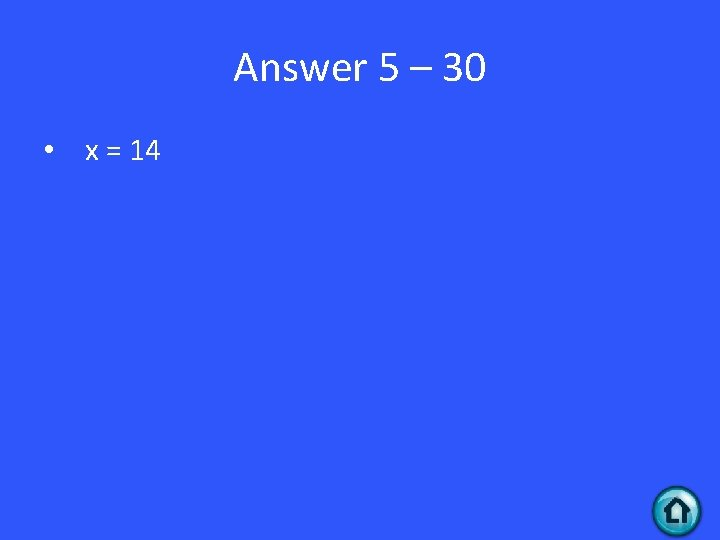Answer 5 – 30 • x = 14Question 5 - 40 • Find the values of the variables:Answer 5 – 40 • x = 5 y = 11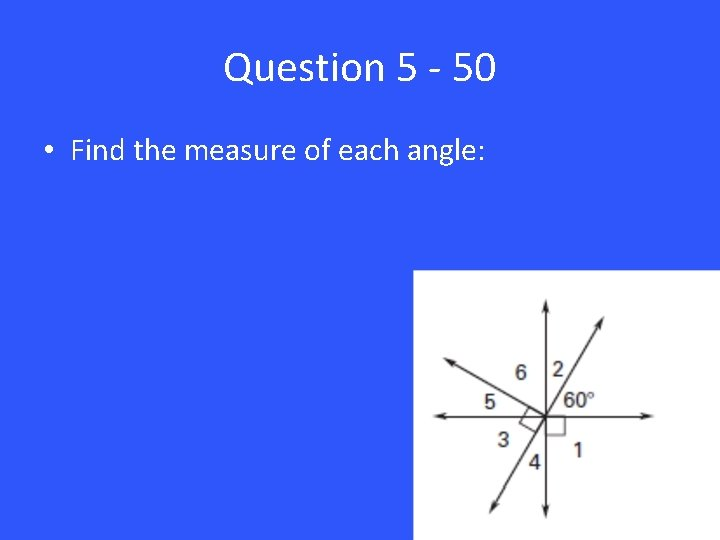Question 5 - 50 • Find the measure of each angle: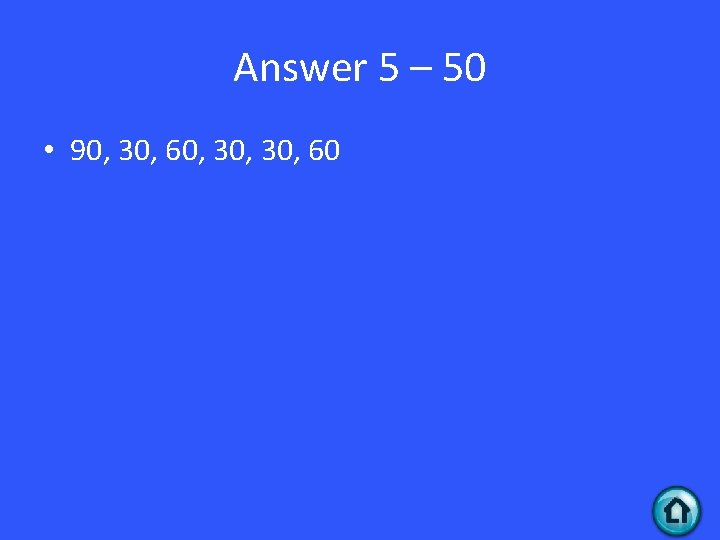Answer 5 – 50 • 90, 30, 60, 30, 60